Science: Physics:
Hydraulic Resistance of Bodies in Water Flow

By Dr. Alexander B. Shandyba

Sumy State University, Ukraine

ABSTRACT: The paper presents the calculation model for hydraulic resistance of bodies in water flow. Experimental verification is made for the axially symmetric cases. A satisfactory agreement is obtained to confirm the influence of the local attack angle and current cross-sectional area of flow contraction.

1. INTRODUCTION

The conical contraction is the most widespread unit of many technical systems.Also it is the noticeable sample of hydrodynamic interaction between flow and streamlined bodies. In this consideration we shall be limited by the developed turbulence regime that allows us to examine the influence of the contraction geometry, pressure distribution, energy losses and drag resistance.

2. THEORY

It was found that the loss of pressure in axially symmetric conical contraction (fig. 1) is connected with the excess pressure of viscous flow to ideal flow by the following equation: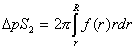���������� (1)

whereis loss of pressure,is lesser cross-sectional area, r, R are radiuses of lesser and greater cross sections, and f(r) is excess pressure of viscous flow to ideal flow.

To determine this function f(r) we suppose the whole flow in the contraction as the complex of elementary streams where pressure and velocity are averaged on time according to the Reynolds-Boussinesqe model [1,5].

Taking into account the change of the flow structure in contraction, one must consider the two characteristic sections of the flow: before and into contraction. The character of the interaction of each stream with the conical surface depends on its initial disposition in flow before contraction and the contraction geometry. At this point of view the boundary streams seem to be most important. Under the unseparated streamlining movement, these have quite defined ways like the contraction formative lines [1,2].

Using the impulse conservation equation the excess pressure can be found for the boundary streams. Thus, if a liquid particle with mass equal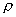has the impulsein cross-section 1-1(where k0 is ratio of boundary stream velocity to average flow velocity before contraction), then its impulse will be equal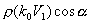after interacting with the conical surface under attack angle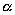.

The corresponding excess pressure in the connection point of conical contraction will be defined from Bernoulli's equation:��������� (2)

where excess pressure function���������������������� (3)��������������

The experimental data confirm the presence and proportionality of the excess pressure to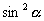function [1,2,5]. It is important to note the increasing of excess pressure along the boundary streams on any head streamlining surface under contraction of flow. This takes place because there is energy redistribution in contraction connected with increasing energy of the boundary streams and accordingly decreasing energy of the inside streams. The corresponding excess pressure occurs due to the change of impulses of the inside streams. The value of pressure change may be found from the following arguments.

First, the excess pressure of real flow to ideal flow is the result of the interaction between flow and the inside surface of contraction. It is connected with the changes of velocities and, accordingly, liquid particles' impulses in the streams. Moreover, only a part of impulse energy is consumed for increasing potential energy of the boundary streams. This increasing conforms tofunction.

From the impulse conservation we can see that interaction of the inside streams with the contraction surface will be analogous with the boundary streams' interaction under their turning.In other words, a ratio of excess potential f and kineticenergy is kept constantly on all inside surfaces of the conical contraction,

i.e., if���,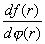=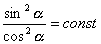������������ 4 )

Generally speaking, the distribution of the excess energy in the boundary streams depends on the initial impulses distribution in flow and the local angles of interaction with the contraction surface. The excess energy distribution can be expressed as the sum:����������������� (5)

Second, the velocity of considering streams will increase in accordance with reduction of cross-sections of the contraction as well as the excess pressure will increase proportionally to the contraction degree function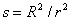. It is very essential that the summary increase of kinetic energy of the boundary streams consists of the ideal and impulse components. The impulse component is increased by energy reduction of the inside streams having the liquid particles with rkV1 impulses, where k function increases from k0 to kmax for axis.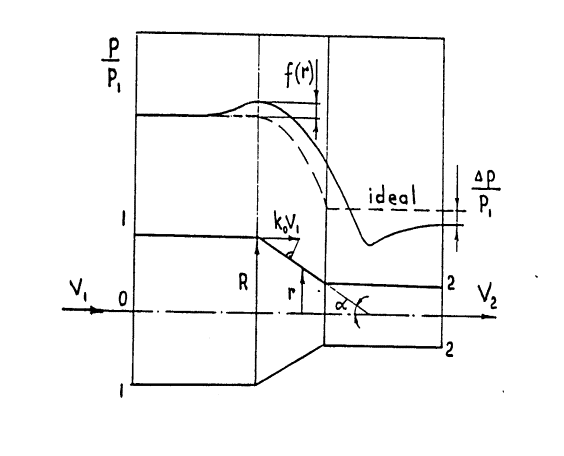Figure 1.Excess pressure of viscous flow to ideal flow

At the same time, the ideal component is increased by Bernoulli's equation and correlated to R4 /r4 function in accordance with the continuous equation. By the same reason, the summary increase of kinetic energy of the boundary streams also is correlated to this contraction degree function [6,7].

Evidently, the impulse kinetic component dj is changed as the difference in analogous way:������� (6)

Therefore, from eq. (5) the excess pressure will depend on this function too: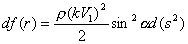������������������ (7)

As integral, the excess pressure distribution on inside surface of contraction is:�������� (8)

The shape component of drag resistance force can be defined as:������������ (9)

3. AXIALLY SYMMETRIC CASE

Flattening the k function can be observed under a sufficiently large Reynolds number and even profile of velocity before contraction. But these conditions are characteristic rather for the external streamlining. In this case we shall consider a cylindrical body with conical head situated in tube (fig.2).

Obviously, we can note the peculiar ring contraction at the conical head. Then the hydraulic losses coefficient of the whole body xx may be presented as the sum: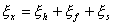������������� (10)

where xh xf xs are the hydraulic loss coefficients of the ring contraction, cylindrical surface friction and Borda's sudden expansion after stern.

Assuming, k = 1 on the analogy of (9) results in:������������������������������ (11)

or after integrating: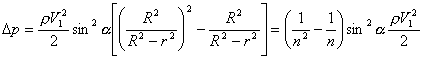������������������ (12)

where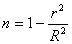,For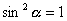��we have the widespread experimental Idelchik's formula: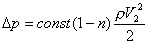������ (13)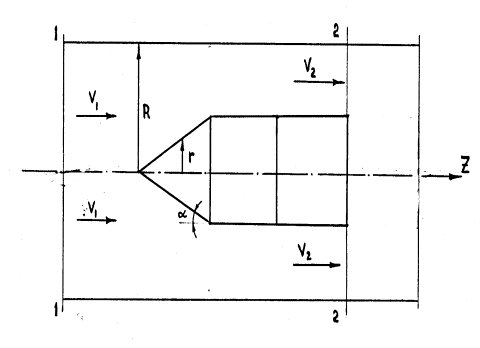Figure 2. Ring contraction at the conical head

From Borda's formula: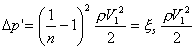������ (14)

Then the shape component of drag resistance force for a short cylinder with a conical head disregarding of friction xf= 0 can be expressed as :������������� (15)

Table 1 gives the comparison between calculated and experimental data of drag resistance coefficient for different contraction degree and free flow.

Table 1. Influence of taper angle and ratio of cross-sectional areas

on drag resistance coefficient (calculation/experiment)

 n Taper angle of head 60o 90o 120o 180o 0.1 103.5/102 126/125 148.5/146 171/174 0.2 21/20 26/26 31/30 36/37 0.3 7.4/7.2 9.3/9.5 11.3/11.5 13.2/13.0 0.4 3.2/3.3 4.1/4.2 5.1/4.9 6.0/6.0 0.5 1.5/1.6 2.0/2.0 2.5/2.4 3.0/2.9 0.6 0.72/0.7 1.0/0.98 1.3/1.3 1.56/1.60 0.695 0.35/0.39 0.51/0.52 0.67/0.67 0.82/0.83 Free flow (exper.) 0.38 0.52 0.66 0.82

4. SYMMETRIC 2D CASE

It can be shown that drag resistance of pier head in stream channel with sufficient depth H on the analogy of (9) is:���������� (16)

Assuming a= const, k = 1, xf = 0 , we obtain the analogous of (15) expression: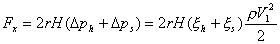�������� (17)

or: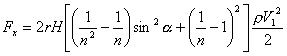������ (18)

where.

At the same time, it is important to see that there is a possibility to reduce the drag resistance by improving shape of pier head (fig.3). Engaging by the new dimensionless coordinates:����������� (19)

we have the boundary conditions: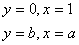����������� (20)

��������������������

and thefunction as: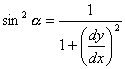������������ (21)

Thereby, in the accepted coordinates system for k = const, the optimal shape of pier head must provide the minimum of the integral:��������������� (22)

In other words, the Euler's differential equation must be executed:��������������� (23)

But this means that the differentiating expression is constant C. Involving the positive

parameter��we obtain the equations system: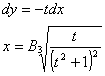���������������� (24)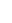whereAfter transformation:����������� (25)

Thus, we have the parameter model for the convex curves family with reducing curvature along flow. For the right solution one must employ the boundary conditions (20).

5. CONCLUDING REMARKS

The results shown in the present paper concern mainly water flow but may be used for the calculation of lifting force and Lilienthal polar of asymmetric bodies in 2D/3D airflow. The main problem of the proposed approach is to identify the contraction degree function correctly, defined only for inside problem of hydroaerodynamics.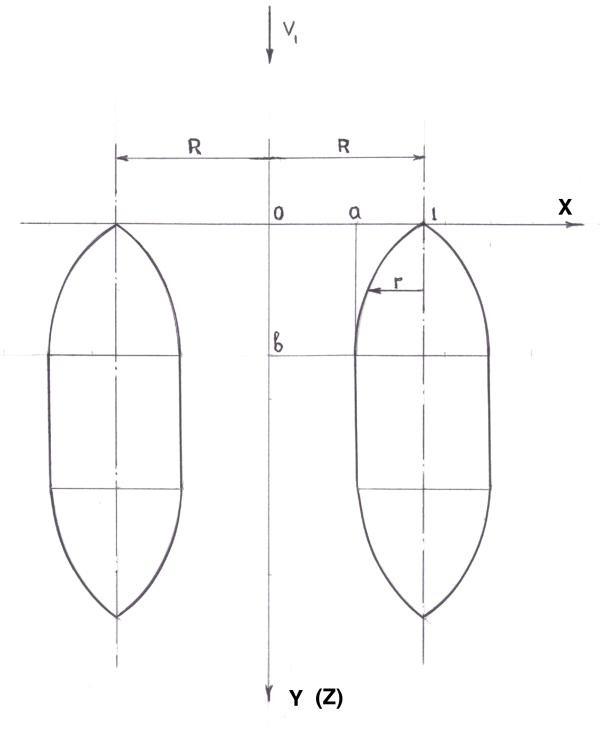Figure 3. Improving shape of pier head

REFERENCES:

1. Daily J., Harleman D., 1971, "Fluid Mechanics", Energy Book Comp. Moscow (transl. from English).

2. Gurevich M.I., 1979, "Theory of jets in ideal fluids", Nauka Publish. Inc/, Moscow (in Russian).

3. Wieghardt T. "Uber Antriebsverteilung des einfachen Rechteckflugel uber die Tiefe", ZAMM, 1939, Bd 19, H.5.

4. Relf E.F., Powell C.H."Tests on smooth and stranded wires inclined to the wind direction", Rep. N 307, ACA, 1916/17.

5. Shandyba A.B. "Hydraulic Resistance of Conical Contraction", in "Collection of articles on Chemical Machine-building", Kiev, 1992, pp. 89-94 (in Russian).

6. Shandyba A.B. "Pressure distribution on head surface of axially symmetric bodies", - Proc. of the "Research and Education - 98 Conference, April 8-9, Sumy, 12 pp., 1998.

7."Numerical Methods in Fluid Dynamics". Ed: Wirz H.J., Smolderen J.J.-von Karman Institute for Fluid Dynamics, Hemisphere Publ. Corp.

Dr. Alexander Shandyba is Assistant Professor of Safety Vital Activity at Sumy State University and heads a research group at New Technology Institute. He has devised numerous projects and is the author of 60 technical papers and inventions. Dr. Shandyba is also a Member of the publishing board of the Mechanical Engineering Journal.

[ back to "Publications & Special Reports" ]
[ BWW Society Home Page ]